omG8HI9LSKFW6lhCj8prTs0Z6lUhdOT9Jhi1Sf4m

# RMS Voltage and Current for AC Circuits

The idea of effective value arises from the need to measure the effectiveness of a voltage or current source in delivering power to a resistive load.
The effective value of a periodic current is the dc current that delivers the same average power to a resistor as the periodic current.
Make sure to read what is ac circuit first.

## How to Calculate Root Mean Square Value

In Figure.(1), the circuit in (a) is ac while the circuit in (b) is dc. Our objective is to find Ieff that will transfer the same power to resistor R as the sinusoid i.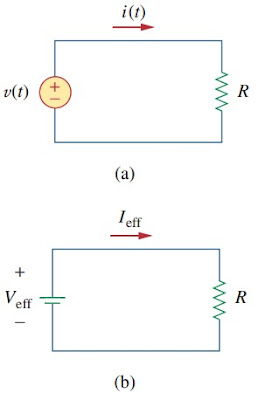Figure 1. Finding the effective current: (a) ac circuit, (b) dc circuit
The average power absorbed by the resistor in the ac circuit is(1)
while the power absorbed by the resistor in the dc circuit is(2)
Equating the expressions in Equations.(1) and (2) and solving for Ieff, we obtain(3)
The effective value of the voltage is found in the same way as current; that is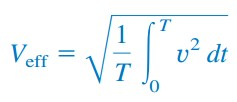(4)
This indicates that the effective value is the (square) root of the mean (or average) of the square of the periodic signal. Thus, the effective value is often known as the root-mean square value, or rms value for short; and we write(5)
For any periodic function x(t) in general, the rms value is given by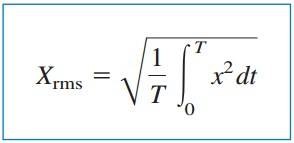(6)
The effective value of a periodic signal is its root mean square (rms) value.
Equation.(6) states that to find the rms value of x(t), we first find its square x2 and then find the mean of that, or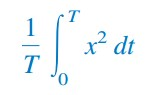(7)
and the square root (√) of that mean. The rms value of a constant is the constant itself. For the sinusoid i(t)Im cos ωt, the effective or rms value is(8)
Similarly, for v(t)Vm cos ωt,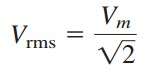(9)
Keep in mind that Equations.(8) and (9) are only valid for sinusoidal signals.

Before moving on, remember all the equations we had in Instantaneous Power and Average Power Formula.
The average power can be written in terms of the rms values(10)
Similarly, the average power absorbed by a resistor R can be written as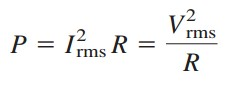(11)
When a sinusoidal voltage or current is specified, it is often in terms of its maximum (or peak) value or its rms value, since its average value is zero. The power industries specify phasor magnitudes in terms of their rms values rather than peak values. For instance, the 110 V available at every household is the rms value of the voltage from the power company.

It is convenient in power analysis to express voltage and current in their rms values. Also, analog voltmeters and ammeters are designed to read directly the rms value of voltage and current, respectively.

## Root Mean Square Value Examples

For better understanding let us review examples below :
1. Determine rms value of the current waveform in Figure.(2). If the current is passed through a 2 Ω resistor, find the average power absorbed by the resistor.Figure 2
Solution :
The period of the waveform is T = 4. Over a period, we can write the current waveform as
The rms value is
The power absorbed by a 2 Ω resistor is

2. The waveform is shown in Figure.(3) is a half-wave rectified sine wave. Find the rms value and the amount of average power dissipated in a 10 Ω resistor.Figure 3
Solution :
The period of the voltage waveform is T = 2π, and
The rms value is obtained as
But sin2t = ½(1 - cos 2t). Hence,
The average power absorbed is

Have you understood what is root mean square? Don't forget to share and subscribe! Happy learning!
Reference:  Fundamentals of electric circuits by Charles K. Alexander and Matthew N. O. Sadiku

Untuk Bahasa Indonesia baca Rumus Nilai Efektif Atau RMS.
Related Posts
SHARE